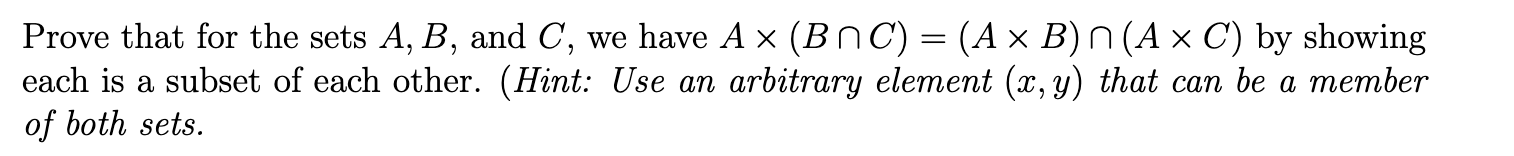Home / Answered Questions / Other / prove-that-for-the-sets-a-b-and-c-we-have-a-bnc-a-x-b-n-a-x-c-by-showing-each-is-a-subset-of-each-ot-aw545

# (Solved): Prove That For The Sets A, B, And C, We Have A ~ (BNC) = (A X B) N(A X C) By Showing Each Is A Subse...Prove that for the sets A, B, and C, we have A ~ (BNC) = (A x B) n(A x C) by showing each is a subset of each other. (Hint: Use an arbitrary element (x, y) that can be a member of both sets.

We have an Answer from Expert